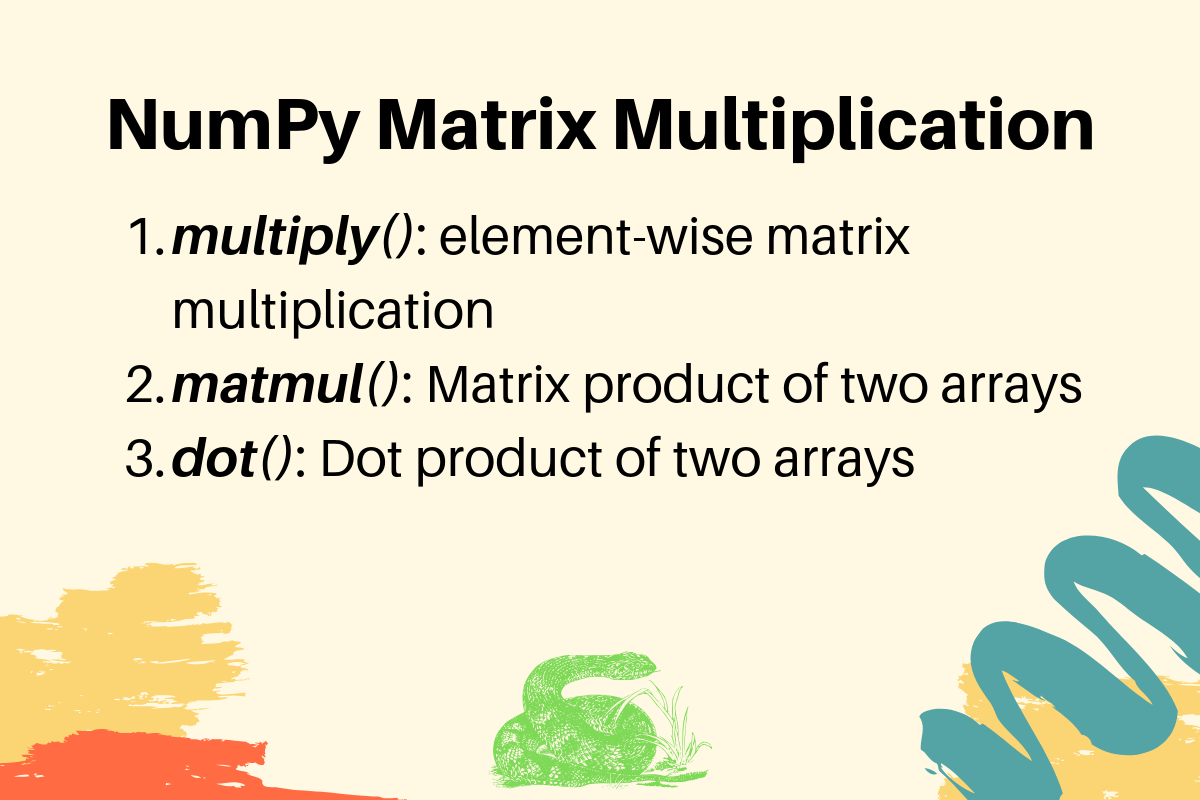# NumPy Matrix Multiplication

Published on August 3, 2022By PankajWhile we believe that this content benefits our community, we have not yet thoroughly reviewed it. If you have any suggestions for improvements, please let us know by clicking the “report an issue“ button at the bottom of the tutorial.

NumPy matrix multiplication can be done by the following three methods.

1. multiply(): element-wise matrix multiplication.
2. matmul(): matrix product of two arrays.
3. dot(): dot product of two arrays.

## 1. NumPy Matrix Multiplication Element Wise

If you want element-wise matrix multiplication, you can use multiply() function.

``````import numpy as np

arr1 = np.array([[1, 2],
[3, 4]])
arr2 = np.array([[5, 6],
[7, 8]])

arr_result = np.multiply(arr1, arr2)

print(arr_result)
``````

Output:

``````[[ 5 12]
[21 32]]
``````

The below image shows the multiplication operation performed to get the result matrix.

## 2. Matrix Product of Two NumPy Arrays

If you want the matrix product of two arrays, use matmul() function.

``````import numpy as np

arr1 = np.array([[1, 2],
[3, 4]])
arr2 = np.array([[5, 6],
[7, 8]])

arr_result = np.matmul(arr1, arr2)

print(f'Matrix Product of arr1 and arr2 is:\n{arr_result}')

arr_result = np.matmul(arr2, arr1)

print(f'Matrix Product of arr2 and arr1 is:\n{arr_result}')
``````

Output:

``````Matrix Product of arr1 and arr2 is:
[[19 22]
[43 50]]
Matrix Product of arr2 and arr1 is:
[[23 34]
[31 46]]
``````

The below diagram explains the matrix product operations for every index in the result array. For simplicity, take the row from the first array and the column from the second array for each index. Then multiply the corresponding elements and then add them to reach the matrix product value.

The matrix product of two arrays depends on the argument position. So matmul(A, B) might be different from matmul(B, A).

## 3. Dot Product of Two NumPy Arrays

The numpy dot() function returns the dot product of two arrays. The result is the same as the matmul() function for one-dimensional and two-dimensional arrays.

``````import numpy as np

arr1 = np.array([[1, 2],
[3, 4]])
arr2 = np.array([[5, 6],
[7, 8]])

arr_result = np.dot(arr1, arr2)

print(f'Dot Product of arr1 and arr2 is:\n{arr_result}')

arr_result = np.dot(arr2, arr1)

print(f'Dot Product of arr2 and arr1 is:\n{arr_result}')

arr_result = np.dot([1, 2], [5, 6])
print(f'Dot Product of two 1-D arrays is:\n{arr_result}')
``````

Output:

``````Dot Product of arr1 and arr2 is:
[[19 22]
[43 50]]
Dot Product of arr2 and arr1 is:
[[23 34]
[31 46]]
Dot Product of two 1-D arrays is:
17
``````

Click below to sign up and get \$200 of credit to try our products over 60 days!

### Popular Topics##### Hollie's Hub for Good

Working on improving health and education, reducing inequality, and spurring economic growth? We’d like to help.##### Become a contributor

You get paid; we donate to tech nonprofits.

##### Welcome to the developer cloud

DigitalOcean makes it simple to launch in the cloud and scale up as you grow – whether you’re running one virtual machine or ten thousand.© 2023 DigitalOcean, LLC.